Home | | Compiler Design | Finding Synchronization-Free Parallelism

# Finding Synchronization-Free Parallelism

1 An Introductory Example 2 Affine Space Partitions 3 Space-Partition Constraints 4 Solving Space-Partition Constraints 5 A Simple Code-Generation Algorithm 6 Eliminating Empty Iterations 7 Eliminating Tests from Innermost Loops 8 Source-Code Transforms 9 Exercises for Section 11.7

Finding Synchronization-Free Parallelism

1 An Introductory Example

2 Affine Space Partitions

3 Space-Partition Constraints

4 Solving Space-Partition Constraints

5 A Simple Code-Generation Algorithm

6 Eliminating Empty Iterations

7 Eliminating Tests from Innermost Loops

8 Source-Code Transforms

9 Exercises for Section 11.7

Having developed the theory of affine array accesses, their reuse of data, and the dependences among them, we shall now begin to apply this theory to paral-lelization and optimization of real programs. As discussed in Section 11.1.4, it is important that we find parallelism while minimizing communication among processors. Let us start by studying the problem of parallelizing an application without allowing any communication or synchronization between processors at all. This constraint may appear to be a purely academic exercise; how often can we find programs and routines that have such a form of parallelism? In fact, many such programs exist in real life, and the algorithm for solving this problem is useful in its own right. In addition, the concepts used to solve this problem can be extended to handle synchronization and communication.

1. An Introductory Example

Shown in Fig. 11.23 is an excerpt of a C translation (with Fortran-style array accesses retained for clarity) from a 5000-line Fortran multigrid algorithm to solve three-dimensional Euler equations.  The program spends most its time in a small number of routines like the one shown in the figure. It is typical of many numerical programs. These often consist of numerous for-loops, with different nesting levels, and they have many array accesses, all of which are affine expressions of surrounding loop indexes. To keep the example short, we have elided lines from the original program with similar characteristics.

The code of Fig. 11.23 operates on the scalar variable T and a number of different arrays with different dimensions. Let us first examine the use of variable T. Because each iteration in the loop uses the same variable T, we cannot execute the iterations in parallel. However, T is used only as a way to hold a common subexpression used twice in the same iteration. In the first two of the three loop nests in Fig. 11.23, each iteration of the innermost loop writes a value into T and uses the value immediately after twice, in the same iteration. We can eliminate the dependences by replacing each use of T by the right-hand-side expression in the previous assignment of T, without changing the semantics of the program. Or, we can replace the scalar T by an array. We then have each iteration use its own array element T[j, i].

With this modification, the computation of an array element in each as-signment statement depends only on other array elements with the same values for the last two components (j and i, respectively). We can thus group alloperations that operate on the (j,i)th element of all arrays into one computa-tion unit, and execute them in the original sequential order. This modification produces ( j l — 1) x ( i l — 1) units of computation that are all independent of one another. Notice that second and third nests in the source program involve a third loop, with index k. However, because there is no dependence between dynamic accesses with the same values for j and «, we can safely perform the loops on k inside the loops on j and i — that is, within a computation unit.

Knowing that these computation units are independent enables a number of legal transforms on this code. For example, instead of executing the code as originally written, a uniprocessor can perform the same computation by execut-ing the units of independent operation one unit at a time. The resulting code, shown in Fig. 11.24, has improved temporal locality, because results produced are consumed immediately.

The independent units of computation can also be assigned to different processors and executed in parallel, without requiring any synchronization or communication. Since there are ( j l — 1) x ( i l — 1) independent units of com putation, we can utilize at most ( j l - 1) x ( i l - 1) processors. By organizing the processors as if they were in a 2-dimensional array, with ID's where 2  < j  <  jl  and  2  <  i  <  i l , the  SPMD program to be executed by  each processor is simply the body in the inner loop in Fig. 11.24.The above example illustrates the basic approach to finding synchronization-free parallelism. We first split the computation into as many independent units as possible. This partitioning exposes the scheduling choices available. We then assign computation units to the processors, depending on the number of processors we have. Finally, we generate an SPMD program that is executed on each processor.

2. Affine Space Partitions

A loop nest is said to have k degrees of parallelism if it has, within the nest, k parallelizable loops — that is, loops such that there are no data dependencies between different iterations of the loops. For example, the code in Fig. 11.24 has 2 degrees of parallelism. It is convenient to assign the operations in a com-putation with k degrees of parallelism to a processor array with k dimensions.

We  shall  assume initially that each  dimension of the processor array has as many processors as there are iterations of the corresponding loop.  After all the independent computation units have been found, we shall map these "virtual" processors to the actual processors. In practice, each processor should be responsible for a fairly large number of iterations, because otherwise there is not enough work to amortize away the overhead of parallelization.

We break down the program to be parallelized into elementary statements, such as 3-address statements. For each statement, we find an affine space partition that maps each dynamic instance of the statement, as identified by its loop indexes, to a processor ID.

Example 1 1 . 4 0 : As discussed above, the code of Fig. 11.23 has two degrees of parallelism. We view the processor array as a 2-dimensional space. Let (pi,P2) be the ID of a processor in the array. The parallelization scheme discussed in Section 11.7.1 can be described by simple affine partition functions. All the statements in the first loop nest have this same affine partition:All the statements in the second and third loop nests have the following same affine partition:The algorithm to find synchronization-free parallelism consists of three steps:

1.          Find, for each statement in the program, an affine partition that maxi-mizes the degree of parallelism. Note that we generally treat the state-ment, rather than the single access, as the unit of computation. The same affine partition must apply to each access in the statement. This grouping of accesses makes sense, since there is almost always dependence among accesses of the same statement anyway.

2.          Assign the resulting independent computation units among the processors, and choose an interleaving of the steps on each processor. This assignment is driven by locality considerations.

3.          Generate an SPMD program to be executed on each processor.

We shall discuss next how to find the affine partition functions, how to gen-erate a sequential program that executes the partitions serially, and how to generate an SPMD program that executes each partition on a different pro-cessor. After we discuss how parallelism with synchronizations is handled in Sections 11.8 through 11.9.9, we return to Step 2 above in Section 11.10 and discuss the optimization of locality for uniprocessors and multiprocessors.

3. Space-Partition Constraints

To require no communication, each pair of operations that share a data depen-dence must be assigned to the same processor. We refer to these constraints as "space-partition constraints." Any mapping that satisfies these constraints cre-ates partitions that are independent of one another. Note that such constraints can be satisfied by putting all the operations in a single partition. Unfortu-nately, that "solution" does not yield any parallelism. Our goal is to create as many independent partitions as possible while satisfying the space-partition constraints; that is, operations are not placed on the same processor unless it is necessary.

When we restrict ourselves to affine partitions, then instead of maximizing the number of independent units, we may maximize the degree (number of dimensions) of parallelism. It is sometimes possible to create more independent units if we can use piecewise affine partitions. A piecewise affine partition divides instances of a single access into, different sets and allows a different affine partition for each set. However, we shall not consider such an option here.

Formally, an affine partition of a program is  synchronization free if and only if for every two (not necessarily distinct) accesses sharing a dependence, T1  = ( F 1 , f 1 , B 1 , b 1 ) in statement s1 nested in d1  loops, and T2 = ( F 2 , f y , B 2 , b 2 ) in statement s2    nested in d2    loops, the partitions ( C 1 , c 1 ) and ( C 2 , c 2 ) for statements si and s 2 , respectively, satisfy the  following  space-partition  constraints: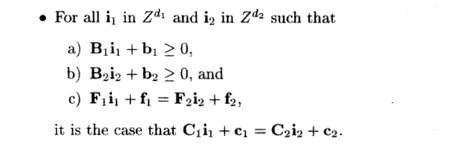The goal of the parallelization algorithm is to find, for each statement, the partition with the highest rank that satisfies these constraints.

Shown in Fig. 11.25 is a diagram illustrating the essence of the space-partition constraints. Suppose there are two static accesses in two loop nests with index vectors ii and i 2 . These accesses are dependent in the sense that they access at least one array element in common, and at least one of them is a write. The figure shows particular dynamic accesses in the two loops that hap pen to access the same array element, according to the affine access functions Fiii + fi and F 2 i 2  +f 2 . Synchronization is necessary unless the affine partitions for the two static accesses, C i i i + c i and C 2 i 2 + c 2 , assign the dynamic accesses to the same processor.

If we choose an affine partition whose rank is the maximum of the ranks of all statements, we get the maximum possible parallelism. However, under this partitioning some processors may be idle at times, while other processors are executing statements whose affine partitions have a smaller rank. This situation may be acceptable if the time taken to execute those statements is relatively short. Otherwise, we can choose an affine partition whose rank is smaller than the maximum possible, as long as that rank is greater than 0.

We show in Example 11.41 a small program designed to illustrate the power of the technique. Real applications are usually much simpler than this, but may have boundary conditions resembling some of the issues shown here. We shall use this example throughout this chapter to illustrate that programs withaffine accesses have relatively simple space-partition constraints, that these con-straints can be solved using standard linear algebra techniques, and that the desired SPMD program can be generated mechanically from the affine parti-tions.

Example 1 1 . 4 1 : This example shows how we formulate the space-partition constraints for the program consisting of the small loop nest with two state-ments, si and s2, shown in Figure 11.26.We show the data dependences in the program in Figure 1L27. That is, each black dot represents an instance of statement Si, and each white dot represents a n instance o f statement S 2 . The dot located a t coordinates (i, j ) represents the instance of the statement that is executed for those values of the loop indexes.

Note, however, that the instance of s2 is located just below the instance of «i for the same pair, so the vertical scale of j is greater than the horizontal scale of i.

Notice that X[i,j] is written by si (i, j), that is, by the instance of statement si with index values i and j. It is later read by s2(i,j + 1), so si(i,j) must precede s2(i,j + 1). This observation explains the vertical arrows from black dots to white dots. Similarly, Y[i,j] is written by s2(i,j) and later read by s1(i + 1, j). Thus, s2(i,j) must precede si(i + l,j), which explains the arrows from white dots to black.It is easy to see from this diagram that this code can be parallelized without synchronization by assigning each chain of dependent operations to the same processor. However, it is not easy to write the SPMD program that implements this mapping scheme. While the loops in the original program have 100 itera-tions each, there are 200 chains, with half originating and ending with statement si and the other half originating and ending with s2. The lengths of the chains vary from 1 to 100 iterations.

Since there are two statements, we are seeking two affine partitions, one for each statement. We only need to express the space-partition constraints for one-dimensional affine partitions. These constraints will be used later by the solution method that tries to find all the independent one-dimensional affine partitions and combine them to get multidimensional affine partitions. We can thus represent the affine partition for each statement by a 1 x 2 matrix and a 1 x 1

vector to translate the vector of indexes into a single processor number. Let ([C11C12], [ci]), ([C2iC22], [c2 ]), be the one-dimensional affine partitions for the statements s1   and s2, respectively.  We apply six data dependence tests:

1. Write  access  X[i,j]  and itself in statement  si,

2. Write access X[i,j]  with read access X[i, j]  in  statement  si,

3. Write access X[i,j] in statement si with read access X[i,j — 1] in state-ment 82,

4. Write access ^ [ i , j] and itself in statement s2,

5. Write access Y[i,j] with read access Y[i,j] in statement s2,

6. Write access Y[i, j] in statement s2  with read access Y[i — l,j] in statement si .

We see that the dependence tests are all simple and highly repetitive. The only dependences present in this code occur in case (3) between instances of accesses

j] and X[i,j — 1] and in case (6) between Y[i,j] and Y[i — 1, j ] .

The space-partition constraints imposed by the data dependence between

X[i,j]  in statement si and X[i,j — 1]  in statement  s2 can be expressed in the following terms:

For all and  such that

1 < i < 100 1 < j < 100

1 < i' < 100 1 < j ' < 100

i = i'

we haveThat is, the first four conditions say that  (i,j) and  lie within the iteration space of the loop nest, and the last two conditions say that the dynamic accesses X[i, j] and X[i,j — 1]  touch the same array element.  We can derive the space-partition constraint for accesses Y[i — in statement s2 and Y[i, j] in statement si in a similar manner.

4. Solving Space-Partition Constraints

Once the space-partition constraints have been extracted, standard linear alge-bra techniques can be used to find the affine partitions satisfying the constraints. Let us first show how we find the solution to Example 11.41.

Example 11 . 42 : We can find the affine partitions for Example 11.41 with the following steps:

1.          Create the space-partition constraints shown in Example 11.41. We use the loop bounds in determining the data dependences, but they are not used in the rest of the algorithm otherwise.3. The equation above holds for all combinations of t1 and t2- Thus, it must be that4.          Find all the independent solutions to the equations involving only un-knowns in the coefficient matrix, ignoring the unknowns in the constant vectors in this step. There is only one independent choice in the coef-

ficient matrix, so the affine partitions we seek can have at most a rank of one. We keep the partition as simple as possible by setting Cn — 1. We cannot assign 0 to C11 because that will create a rank-0 coefficient matrix, which maps all iterations to the same processor. It then follows that C2i = 1, C22 = - 1, C12 = - 1 .

5. Find the constant terms. We know that the difference between the constant terms, c2 — ci, must be — 1 . We get to pick the actual values, however. To keep the partitions simple, we pick c2 = 0; thus ci = — 1 .

Let p be the ID of the processor executing iteration In terms of p, the affine partition isThat is, the (i,j)th iteration of si is assigned to the processor p = i — j — 1, and the (i,j)th iteration of s2 is assigned to processor p — % — j.

Algorithm 11.43 : Finding a highest-ranked synchronization-free affine partition for a program.

INPUT : A program with affine array accesses.

OUTPUT : A partition.

METHOD : Do the following:

1.  Find all data-dependent pairs of accesses in a program for each pair of data-dependent accesses, T1  — ( F i , f i , B i , b i ) in statement s1  nested in di  loops  and T2 =  ( F 2 , f 2 , B 2  , b 2  ) in  statement  s2    nested  in  d2    loops. Let ( C i , c i ) and ( C 2 , c 2 ) represent the (currently unknown) partitions of statements s1  and s2, respectively. The space-partition constraints statefor all i1 and i2, within their respective loop bounds. We shall generalize the domain of iterations to include all ii in Zdl and i2 in Zd2; that is, the bounds are all assumed to be minus infinity to infinity. This assumption makes sense, since an affine partition cannot make use of the fact that an index variable can take on only a limited set of integer values.

2. For each pair of dependent accesses, we reduce the number of unknowns in the index vectors.

(a)  Note that Fi + f is the same vector as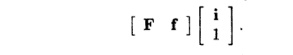That is, by adding an extra component 1 at the bottom of column-vector i, we can make the column-vector f be an additional, last column of the matrix F. We may thus rewrite the equality of the access functions(b) The above equations will in general have more than one solution. However, we may still use Gaussian elimination to solve the equations for the components of ix and i2 as best we can. That is, eliminate as many variables as possible until we are left with only variables that cannot be eliminated. The resulting solution for i2 and i2 will have the form where U is an upper-triangular matrix and t is a vector of free vari-ables ranging over all integers.

(c) We may use the same trick as in Step (2a) to rewrite the equality of the partitions. Substituting the vector (ii, i2 ,1) with the result from Step (2b), we can write the constraints on the partitions as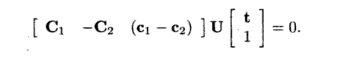3. Drop the nonpartition variables. The equations above hold for all combi-nations of t ifRewrite these equations in the form Ax = 0, where x is a vector of all the unknown coefficients of the affine partitions.

4.          Find the rank of the affine partition and solve for the coefficient matrices. Since the rank of an affine partition is independent of the value of the constant terms in the partition, we eliminate all the unknowns that come

from the constant vectors like ci or c 2 , thus replacing Ax = 0 by sim-plified constraints A'x' = 0. Find the solutions to A'x' = 0, expressing them as B, a set of basis vectors spanning the null space of A'.

5.          Find the constant terms. Derive one row of the desired affine partition from each basis vector in B, and derive the constant terms using Ax = 0.

Note that Step 3 ignores the constraints imposed by the loop bounds on variables t. The constraints are only stricter as a result, and the algorithm must therefore be safe. That is, we place constraints on the C's and c's assuming t is arbitrary. Conceivably, there would be other solutions for the C's and c's that are valid only because some values of t are impossible. Not searching for these other solutions may cause us to miss an optimization, but cannot cause the program to be changed to a program that does something different from what the original program does.

5. A Simple Code-Generation Algorithm

Algorithm 11.43 generates affine partitions that split computations into inde-pendent partitions. Partitions can be assigned arbitrarily to different proces-sors, since they are independent of one another. A processor may be assigned more than one partition and can interleave the execution of its partitions, as long as operations within each partition, which normally have data dependences, are executed sequentially.

It is relatively easy to generate a correct program given an affine partition. We first introduce Algorithm 11.45, a simple approach to generating code for a single processor that executes each of the independent partitions sequentially. Such code optimizes temporal locality, since array accesses that have several uses are very close in time. Moreover, the code easily can be turned into an SPMD program that executes each partition on a different processor. The code generated is, unfortunately, inefficient; we shall next discuss optimizations to make the code execute efficiently.

The essential idea is as follows. We are given bounds for the index variables of a loop nest, and we have determined, in Algorithm 11.43, a partition for the accesses of a particular statement s. Suppose we wish to generate sequential code that performs the action of each processor sequentially. We create an outermost loop that iterates through the processor IDs. That is, each iteration of this loop performs the operations assigned to a particular processor ID. The original program is inserted as the loop body of this loop; in addition, a test is added to guard each operation in the code to ensure that each processor only executes the operations assigned to it. In this way, we guarantee that the processor executes all the instructions assigned to it, and does so in the original sequential order.

Example 11.44: Let us generate code that executes the independent parti-tions in Example 11.41 sequentially. The original sequential program is from Fig. 11.26 is repeated here as Fig. 11.28.In Example 11.41, the affine partitioning algorithm found one degree of parallelism. Thus, the processor space can be represented by a single variable p. Recall also from that example that we selected an affine partition that, for all values of index variables i and j with 1 =< i =< 100 and 1 =< j =< 100, assignedWe can generate the code in three steps:

1. For each statement, find all the processor IDs participating in the com-putation. We combine the constraints 1 =< i =< 100 and 1 =< j =< 100 with one of the equations p = i - j - 1 or p = i - j, and project away i and j to get the new constraints2. Find all the processor IDs participating in any of the statements.  When

we take the union of these ranges, we get - 1 0 0 =< p =< 99; these bounds

are sufficient to cover all instances of both statements si  and s 2 .

3.          Generate the code to iterate through the computations in each partition sequentially. The code, shown in Fig. 11.29, has an outer loop that iterates through all the partition IDs participating in the computation (line (1)). Each partition goes through the motion of generating the indexes of all the iterations in the original sequential program in lines (2) and (3) so that it can pick out the iterations the processor p is supposed to execute. The tests of lines (4) and (6) make sure that statements s1 and s2 are executed only when the processor p would execute them.

The generated code, while correct, is extremely inefficient. First, even though each processor executes computation from at most 99 iterations, it gen-erates loop indexes for 100 x 100 iterations, an order of magnitude more than necessary. Second, each addition in the innermost loop is guarded by a test, creating another constant factor of overhead. These two kinds of inefficiencies are dealt with in Sections 11.7.6 and 11.7.7, respectively. •Although the code of Fig. 11.29 appears designed to execute on a unipro-cessor, we could take the inner loops, lines (2) through (8), and execute them on 200 different processors, each of which had a different value for p, from —100 to 99. Or, we could partition the responsibility for the inner loops among any number of processors less than 200, as long as we arranged that each processor knew what values of p it was responsible for and executed lines (2) through (8) for just those values of p.

Algorithm 11.45 : Generating code that executes partitions of a program sequentially.

INPUT : A program P with affine array accesses. Each statement s in the program has associated bounds of the form B s i + bs > 0, where i is the vector of loop indexes for the loop nest in which statement s appears. Also associated with statement s is a partition C 5 i + cs = p where p is an m-dimensional vector of variables representing a processor ID; m is the maximum, over all statements in program P, of the rank of the partition for that statement.

OUTPUT : A program equivalent to P but iterating over the processor space over loop rather than indexes.

M E T H O D : Do the following:

1.         For each statement, use Fourier-Motzkin elimination to project out all the loop index variables from the bounds.

2.         Use Algorithm 11.13 to determine bounds on the partition ID's.

3.  Generate loops, one for each of the m dimensions of processor space.  Let

p = [pi,P2, -- - ,Pm] be the vector of variables for these loops; that is, there is one variable for each dimension of the processor space. Each loop variable pi ranges over the union of the partition spaces for all statements in the program P.

Note that the union of the partition spaces is not necessarily convex. To keep the algorithm simple, instead of enumerating only those partitions that

have a nonempty computation to perform,  set the lower bound of each pi  to

the minimum of all the lower bounds imposed by all statements and the upper bound of each pi to the maximum of all the upper bounds imposed by all statements. Some values of p may thereby have no operations.

The code to be executed by each partition is the original sequential pro-gram. However, every statement is guarded by a predicate so that only those operations belonging to the partition are executed. •

An example of Algorithm 11.45 will follow shortly. Bear in mind, however, that we are still far from the optimal code for typical examples.

6. Eliminating Empty Iterations

We now discuss the first of the two transformations necessary to generate ef-ficient SPMD code. The code executed by each processor cycles through all the iterations in the original program and picks out the operations that it is supposed to execute. If the code has k degrees of parallelism, the effect is that each processor performs k orders of magnitude more work. The purpose of the first transformation is to tighten the bounds of the loops to eliminate all the empty iterations.

We begin by considering the statements in the program one at a time. A statement's iteration space to be executed by each partition is the original itera-tion space plus the constraint imposed by the affine partition. We can generate tight bounds for each statement by applying Algorithm 11.13 to the new iter-ation space; the new index vector is like the original sequential index vector, with processor ID's added as outermost indexes. Recall that the algorithm will generate tight bounds for each index in terms of surrounding loop indexes.

After finding the iteration spaces of the different statements, we combine them, loop by loop, making the bounds the union of those for each statement. Some loops end up having a single iteration, as illustrated by Example 11.46 below, and we can simply eliminate the loop and simply set the loop index to the value for that iteration.

Example 1 1 . 4 6 :  For the loop of Fig.  11.30(a), Algorithm 11.43 will create the affine partition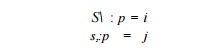Algorithm 11.45 will create the code of Fig. 11.30(b). Applying Algorithm 11.13 to statement s1 produces the bound: p < i < p, or simply % = p. Similarly, the algorithm determines j = p for statement s2- Thus, we get the code of Fig. 11.30(c). Copy propagation of variables i and j will eliminate the unnec-essary test and produce the code of Fig. 11.30(d). •

We now return to Example 11.44 and illustrate the step to merge multiple iteration spaces from different statements together.

Example 11*47:  Let us now tighten the loop bounds of the code in Example 11.44. The iteration space executed by partition p for statement si is defined by the following equalities and inequalities:Applying Algorithm 11.13 to the above creates the constraints shown in Fig. 11.31(a). Algorithm 11.13 generates the constraint p + 2 < i < 100 + p + 1 from i — p - 1 = j and 1 < j < 100, and tightens the upper bound of p to 98.

Likewise, the bounds for each of the variables for statement s2 are shown in Fig. 11.31(b).

The iteration spaces for si and s2 in Fig. 11.31 are similar, but as ex-pected from Fig. 11.27, certain limits differ by 1 between the two. The code in Fig. 11.32 executes over this union of iteration spaces. For example, for i use min(l,£>+1) as the lower bound and max(100,100+ p + 1 ) as the upper bound. Note that the innermost loop has 2 iterations except that it has only one the first and last time it is executed. The overhead in generating loop indexes is thus reduced by an order of magnitude. Since the iteration space executed is larger than either that of si  and  s2, conditionals  are still necessary to select when these statements are executed.7. Eliminating Tests from Innermost Loops

The second transformation is to remove conditional tests from the inner loops. As seen from the examples above, conditional tests remain if the iteration spaces of statements in the loop intersect but not completely. To avoid the need for conditional tests, we split the iteration space into subspaces, each of which executes the same set of statements. This optimization requires code to be duplicated and should only be used to remove conditionals in the inner loops.

To split an iteration space to reduce tests in inner loops, we apply the following steps repeatedly until we remove all the tests in the inner loops:

1. Select a loop that consists of statements with different bounds.

2.          Split the loop using a condition such that some statement is excluded from at least one of its components. We choose the condition from among the boundaries of the overlapping different polyhedra. If some statement has all its iterations in only one of the half planes of the condition, then such a condition is useful.

3. Generate code for each of these iteration spaces separately.

Example 1 1 . 4 8 : Let us remove the conditionals from the code of Fig.  11.32. Statements si  and 52 are mapped to the same set of partition ID's except forThe code for each subspace can then be specialized for the value(s) of p contained. Figure 11.33 shows the resulting code for each of the three iteration

spaces.

Notice that the first and third spaces do not need loops on i or j, because for the particular value of p that defines each space, these loops are degenerate; they have only one iteration. For example, in space (1), substituting p = —100 in the loop bounds restricts i to 1, and subsequently j to 100. The assignments

to p in spaces (1) and (3) are evidently dead code and can be eliminated.

Next we split the loop with index i in space (2). Again, the first and last iterations of loop index i are different. Thus, we split the loop into three subspaces: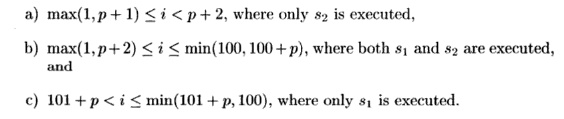The loop nest for space (2) in Fig. 11.33 can thus be written as in Fig. 11.34(a). Figure 11.34(b) shows the optimized program. We have substituted Fig.

11.34(a) for the loop nest in Fig. 11.33. We also propagated out assignments to p, i, and j into the array accesses. When optimizing at the intermediate-code level, some of these assignments will be identified as common subexpressions and re-extracted from the array-access code.8. Source-Code Transforms

We have seen how we can derive from simple affine partitions for each statement programs that are significantly different from the original source. It is not apparent from the examples seen so far how affine partitions correlate with changes at the source level. This section shows that we can reason about source code changes relatively easily by breaking down affine partitions into a series of primitive transforms.

Seven Primitive Affine Transforms

Every affine partition can be expressed as a series of primitive affine transforms, each of which corresponds to a simple change at the source level. There are seven kinds of primitive transforms: the first four primitives are illustrated in Fig. 11.35, the last three, also known as unimodular transforms, are illustrated in Fig. 11.36.

The figure shows one example for each primitive: a source, an affine parti-tion, and the resulting code. We also draw the data dependences for the code before and after the transforms. From the data dependence diagrams, we see that each primitive corresponds to a simple geometric transform and induces a relatively simple code transform. The seven primitives are: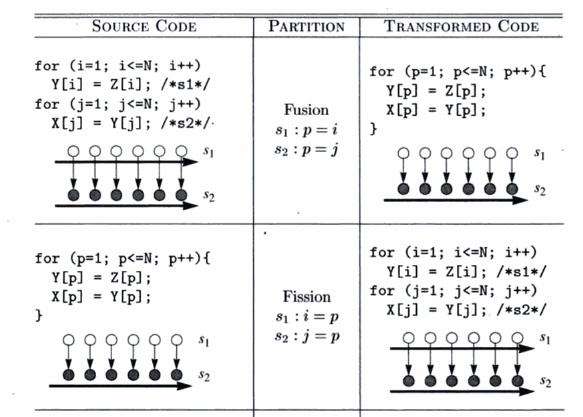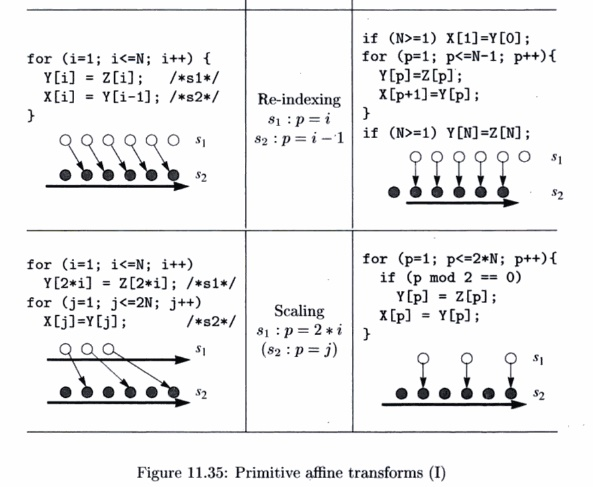Unimodular Transforms

A unimodular transform is represented by just a unimodular coefficient matrix and no constant vector. A unimodular matrix is a square matrix whose determinant is ± 1 . The significance of a unimodular transform is that it maps an n-dimensional iteration space to another n-dimensional polyhedron, where there is a one-to-one correspondence between iterations of the two spaces.

1.          Fusion. The fusion transform is characterized by mapping multiple loop indexes in the original program to the same loop index. The new loop fuses statements from different loops.

2. Fission. Fission is the inverse of fusion. It maps the same loop index for different statements to different loop indexes in the transformed code. This splits the original loop into multiple loops.

3. Re-indexing. Re-indexing shifts the dynamic executions of a statement by a constant number of iterations. The affine transform has a constant term.

4.          Scaling. Consecutive iterations in the source program are spaced apart by a constant factor. The affine transform has a positive nonunit coefficient.

5.         Reversal. Execute iterations in a loop in reverse order. Reversal is char-acterized by having —1 as a coefficient.

6.          Permutation. Permute the inner and outer loops. The affine transform consists of permuted rows of the identity matrix.

7.          Shewing. Iterate through the iteration space in the loops at an angle. The affine transform is a unimodular matrix with l's on the diagonal.

A  Geometric Interpretation  of Parallelization

The affine transforms shown in all but the fission example are derived by apply-ing the synchronization-free affine partition algorithm to the respective source codes. (We shall discuss how fission can parallelize code with synchronization in the next section.) In each of the examples, the generated code has an (outer-most) parallelizable loop whose iterations can be assigned to different processors and no synchronization is necessary.

These examples illustrate that there is a simple geometric interpretation of how parallelization works. Dependence edges always point from an earlier instance to a later instance. So, dependences between separate statements not nested in any common loop follows the lexical order; dependences between statements nested in the same loop follows the lexicographic order. Geometri-cally, dependences of a two-dimensional loop nest always point within the range [0°, 180°), meaning that the angle of the dependence must be below 180°, but no less than 0°.

The affine transforms change the ordering of iterations such that all the dependences are found only between operations nested within the same iteration of the outermost loop. In other words, there are no dependence edges at the boundaries of iterations in the outermost loop. We can parallelize simple source codes by drawing their dependences and finding such transforms geometrically.

9. Exercises for Section 11.7

Exercise 1 1 . 7 . 1 :  For the following loop

for  (i = 2;  i <  100;  i++)

A[i]  =  A[i-2];

a)          What is the largest number of processors that can be used effectively to execute this loop?

b)          Rewrite the code with processor p as a parameter.

c)           Set up and find one solution to the space-partition constraints for this loop.

d)  What is the affine partition of highest rank for this loop?

Exercise 11 . 7 . 2: Repeat Exercise 11.7.1 for the loop nests in Fig. 11.37.

Exercise 11 . 7 . 3:  Rewrite the following code

for (i = 0; i < 100; i++) A[i] = 2*A[i] ;

for (j = 0; j < 100; j++) A[j] = A[j] + 1;

so it consists of a single loop. Rewrite the loop in terms of a processor number p so the code can be partitioned among 100 processors, with iteration p executed by processor p.

Exercise 11 . 7 . 4:  In the following code

for  (i = 1;  i <  100;  i++)

for  (j  = 1;  j  < 100;  j++)

/*  (s)  */   A[i,j]  =

(A[i-l,j]  + A[i+l,j]  + A[i,j-1]  + A[i,j+l])/4;

for (i = 0;  i <= 97;  i++)

A[i] = A[i+2] ;

(a)

for (i = 1;  i <= 100;  i++)

for (j = 1; j <= 100; j++)

for (k = 1;  k <= 100; k++)  {

A[i,j,k]  = A[i,j,k]  + B[i-l,j,k];

B[i,j,k] = B[i,j,k] + C[i, j-l,k] ;

C[i,j,k] = C[i,j,k]  + A[i,j,k-1];

}

!(b)

for (i = 1;  i <= 100;  i++)

for (j = 1; j <= 100; j++)

for (k = 1; k <= 100; k++) {

A[i,j,k]  = A[i,j,k]  + B[i-l,j,k];

B[i,j,k]  = B[i,j,k]  + A[i,j-l,k];

C[i,j,k] = C[i,j,k]  + A[i,j,k-1] + B[i,j,k];

}

!(c)

Figure 11.37: Code for Exercise 11.7.2

the only constraints are that the statement s that forms the body of the loop

nest must execute iterations s(i — l,j) and s(i, j — 1) before executing iteration

s(i,j). Verify that these are the only necessary constraints. Then rewrite the code so that the outer loop has index variable p, and on the pth iteration of the outer loop, all instances of s(i,j) such that i + j = p are executed.

Exercise 1 1 . 7 . 5 : Repeat Exercise 11.7.4, but arrange that on the pth iteration of the outer loop, instances of s such that i — j = p are executed.

! Exercise 1 1 . 7 . 6 : Combine the following loops

for (i = 0; i < 100; i++) A[i] = B[i];

for (j = 98; j >= 0; j = j-2) B[i] = i;

into a single loop, preserving all dependencies.

Exercise 11 . 7 . 7:  Show that the matrixis unimodular. Describe the transformation it performs on a two-dimensional loop nest.

Exercise 11 . 7 . 8:  Repeat Exercise 11.7.7 on the matrixStudy Material, Lecturing Notes, Assignment, Reference, Wiki description explanation, brief detail
Compilers : Principles, Techniques, & Tools : Optimizing for Parallelism and Locality : Finding Synchronization-Free Parallelism |